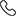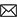Sales :9214233303 | 7240013135 / Tech. Support Desk :7231890000 (9AM ~ 6PM)# Foundation Polynomial for class 10th by MC Sir

Review of polynomial,quadratic equation,cubic polynomial,zero of linear,quadratic,roots/zero/solution of quadratic,linear,geometrical meaning of zerox of polynomial,graph of linear quadratic ,Differentiation/derivative of polynomial,geometric meaning of derivative,slope of any curve,derivative of xn constant function,curve & sketching of polynomial vertex of parabola,condition of max/min zero/roots of polynomial ,Relationship b/w zero and coefficient of linear,Quadratic, Bi-quadratic ,cubic polynomial method of splitting the middle term to find zero of quadratic,identity of linear & quadratic polynomial etc

• Rs.1,000 Rs.1,000
• Rs.2,300 Rs.2,300
• Rs.1,000 Rs.1,000
Subject Medium Target Language Mathematics English X Class English + Hindi Topic Based 8 Videos 0 Videos PDF Files Full coverage of topic Polynomial 10th class Course Structure: Periodic Test is conducted in the form of Part Test & Cumulative Test. Longest time availability as compared to other Courses. Lays solid foundation of basic concepts of all the subjects The focus of the Course is more on concept building & gradual improvement in the performance of the students. Develops quick analytical thinking & systematic problem solving skills consolidate the Mathematical knowledge and skills acquired at the upper primary stage Properly organized solutions in chapter-wise and page wise format help find your solution quickly Must for class 9th and 10th studying students who want to do well in NTSE, Olympiad, KVPY, IIT JEE, Medical and other engineering and competitive exams Ideal for class 10th students who are preparing for NTSE, Olympiad, KVPY, IIT JEE Exams. Essential for higher mathematics learning students. Essential for CBSE, NIOS & ICSE Exam preparations

### Other Courses by Manoj Chauhan SirTap a Star to Rate us:
close
close